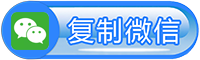# 仿站

## 网站制作实现向两边滑动选项卡菜单

<LI><P><A class=active href="javascript:void(0);">前端开发HTML5</A></P></LI>
<LI><P><A href="javascript:void(0);">前端开发CSS3</A></P></LI>
<P><!–同样的结构，若干个省略掉的li–></P>
<LI><P><A href="javascript:void(0);">前端开发JS</A></P></LI>
<LI><P><A href="javascript:void(0);">WEB前端开发</A></P></LI>
</UL>
<P><!–左右移动按钮–></P>
<P><A class=btn-toLeft href="javascript:void(0);">&lt;&lt;</A></P>
<P><A class=btn-toRight href="javascript:void(0);">&gt;&gt;</A></P>
</DIV>

\$(function () {
var aLi = \$(oTabMenuList).find("li");
var disX = 0;
var l = 0;
function reset() {
var ulWidth = 0;
for (var i = 0; i < aLi.length; i++) {
ulWidth += aLi.eq(i).width() + 10;
}
}
}
function move(dis) {
}
reset();
/*可以添加选项卡的内容，点击相应菜单显示相应内容*/
});
if (l >= -disX) {
l += -100;
move(l);
}
if (l < 0) {
}
if (l < -disX) {
}
});
if (l < 0) {
l += 100;
move(l);
}
if (l >= 0) {
}
if (l >= -disX) {
}
});
});

##### 相似内容

132-80692153